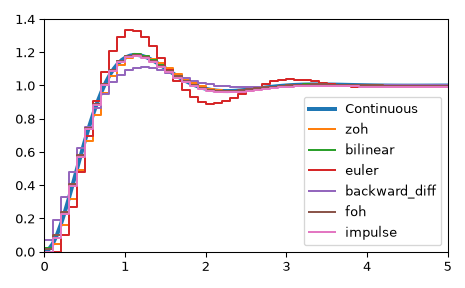# scipy.signal.cont2discrete¶

scipy.signal.cont2discrete(system, dt, method='zoh', alpha=None)[source]

Transform a continuous to a discrete state-space system.

Parameters
systema tuple describing the system or an instance of `lti`

The following gives the number of elements in the tuple and the interpretation:

• 1: (instance of `lti`)

• 2: (num, den)

• 3: (zeros, poles, gain)

• 4: (A, B, C, D)

dtfloat

The discretization time step.

methodstr, optional

Which method to use:

• gbt: generalized bilinear transformation

• bilinear: Tustin’s approximation (“gbt” with alpha=0.5)

• euler: Euler (or forward differencing) method (“gbt” with alpha=0)

• backward_diff: Backwards differencing (“gbt” with alpha=1.0)

• zoh: zero-order hold (default)

• foh: first-order hold (versionadded: 1.3.0)

• impulse: equivalent impulse response (versionadded: 1.3.0)

alphafloat within [0, 1], optional

The generalized bilinear transformation weighting parameter, which should only be specified with method=”gbt”, and is ignored otherwise

Returns
sysdtuple containing the discrete system

Based on the input type, the output will be of the form

• (num, den, dt) for transfer function input

• (zeros, poles, gain, dt) for zeros-poles-gain input

• (A, B, C, D, dt) for state-space system input

Notes

By default, the routine uses a Zero-Order Hold (zoh) method to perform the transformation. Alternatively, a generalized bilinear transformation may be used, which includes the common Tustin’s bilinear approximation, an Euler’s method technique, or a backwards differencing technique.

The Zero-Order Hold (zoh) method is based on , the generalized bilinear approximation is based on  and , the First-Order Hold (foh) method is based on .

References

1

https://en.wikipedia.org/wiki/Discretization#Discretization_of_linear_state_space_models

2

http://techteach.no/publications/discretetime_signals_systems/discrete.pdf

3

G. Zhang, X. Chen, and T. Chen, Digital redesign via the generalized bilinear transformation, Int. J. Control, vol. 82, no. 4, pp. 741-754, 2009. (https://www.mypolyuweb.hk/~magzhang/Research/ZCC09_IJC.pdf)

4

G. F. Franklin, J. D. Powell, and M. L. Workman, Digital control of dynamic systems, 3rd ed. Menlo Park, Calif: Addison-Wesley, pp. 204-206, 1998.

Examples

We can transform a continuous state-space system to a discrete one:

```>>> import matplotlib.pyplot as plt
>>> from scipy.signal import cont2discrete, lti, dlti, dstep
```

Define a continuous state-space system.

```>>> A = np.array([[0, 1],[-10., -3]])
>>> B = np.array([,[10.]])
>>> C = np.array([[1., 0]])
>>> D = np.array([[0.]])
>>> l_system = lti(A, B, C, D)
>>> t, x = l_system.step(T=np.linspace(0, 5, 100))
>>> fig, ax = plt.subplots()
>>> ax.plot(t, x, label='Continuous', linewidth=3)
```

Transform it to a discrete state-space system using several methods.

```>>> dt = 0.1
>>> for method in ['zoh', 'bilinear', 'euler', 'backward_diff', 'foh', 'impulse']:
...    d_system = cont2discrete((A, B, C, D), dt, method=method)
...    s, x_d = dstep(d_system)
...    ax.step(s, np.squeeze(x_d), label=method, where='post')
>>> ax.axis([t, t[-1], x, 1.4])
>>> ax.legend(loc='best')
>>> fig.tight_layout()
>>> plt.show()
```